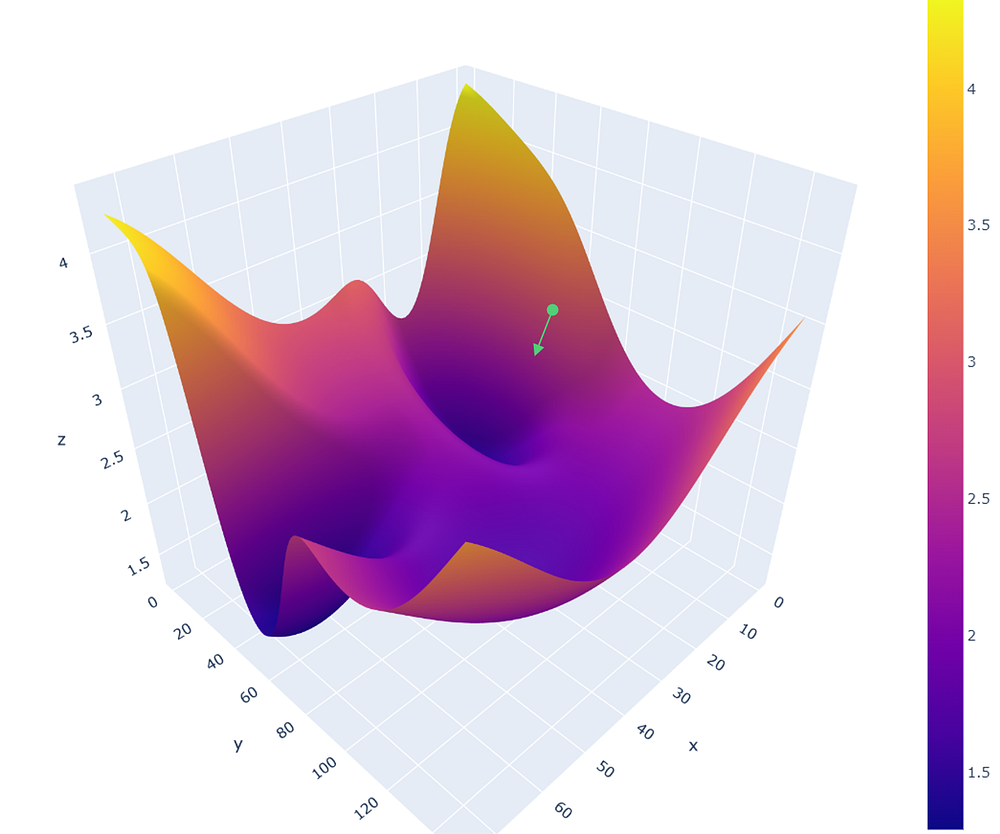# Automatic Differentiation

Source: Deep Learning on Medium# 4. Automatic Differentiation

Autodiff is an elegant approach that can be used to calculate the partial derivatives of any arbitrary function in a given point. It decomposes the function in a sequence of elementary arithmetic operations (+, -, *, /) and functions (max, exp, log, cos, sin…); then uses the chain rule to work out the function’s derivative with respect to its initial parameters.

Note: there are 2 variants of Autodiff:

• Forward-Mode, which is a hybrid of symbolic and numerical differentiation; while numerically precise, it requires one pass through the computational graph for each input parameter, which is resource consuming.
• Reverse-Mode, on the other hand, only requires 2 passes through the computational graph to evaluate both the function and its partial derivatives.

In this post we focus on Reverse-Mode Autodiff, as it is the most popular in practical implementations; for example it is the one used in Tensorflow.

To see how this magic works, let’s start by representing the function f as a computational graph:

There are 2 steps to Reverse-Mode autodiff: a forward pass, during which the function value at the selected point is calculated; and a backward pass, during which the partial derivatives are evaluated.

Forward pass

During the forward pass, the function inputs are propagated down the computational graph:

As expected we get the function value: f(2, 3, 4)=48. We also assigned names to intermediate nodes encountered along the way: x4, x5, x6, x7; they will be used below.

Backward pass

Reverse-Mode autodiff uses the chain rule to calculate the gradient values at point (2, 3, 4).

Let’s calculate the partial derivatives of each node with respect to its immediate inputs:

Note that we can calculate the numerical value of each partial derivative — for example dx5/dx3=x2=3 — thanks to the value for x2 obtained during the forward pass. Also note that the partial derivatives are calculated locally at point (2, 3, 4); should we change the initial point, the derivatives values would also change.

Now that we have the partial derivatives of each node, we can use the chain rule to calculate the partial derivatives of f with respect to its original inputs: x1, x2 and x3.

In calculus, the chain rule is a formula for computing the derivative of the composition of two or more functions:

Remember that we are interested in the following gradient values, evaluated at point (2, 3, 4):